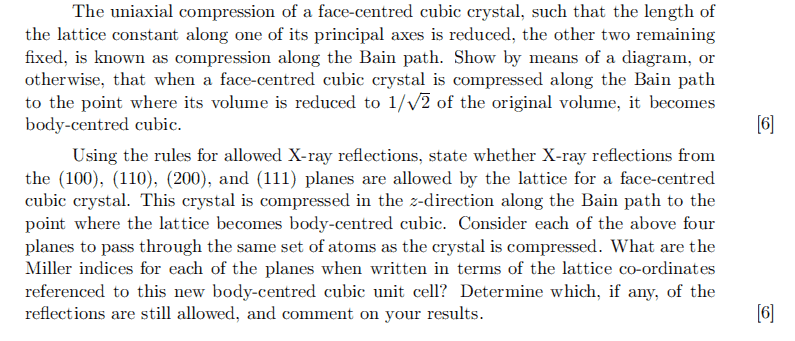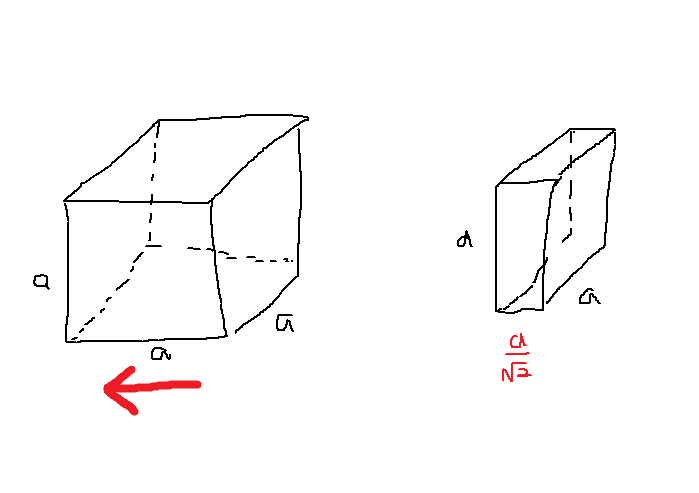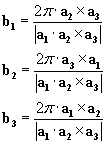# Miller Indices in FCC and BC

unscientific

## Homework Statement

[/B]
(a) Show Compression of FCC leads to BCC.
(b) State rules for X-rays reflection in FCC.
(c) What are the new Miller Indices after compression?## The Attempt at a Solution

Part(a)[/B]
I'm quite confused as to what they mean by 'principal axes'.
For an FCC lattice, the basis is given by ##[0,0,0]## and ##[\frac{1}{2},\frac{1}{2},0]## and ##[\frac{1}{2},0,\frac{1}{2}]## and ##[0,\frac{1}{2},\frac{1}{2}]##..
The primitive lattice vectors are ##\left( \frac{1}{2} \hat x + \frac{1}{2}\hat y + 0 \hat z \right)## and ##\left( \frac{1}{2} \hat x + 0 \hat y + \frac{1}{2} \hat z \right)## and ##\left( 0 \hat x + \frac{1}{2} \hat y + \frac{1}{2} \hat z \right)##.
Are the principal axes the ##x,y,z## axes?

I'm not sure how the Bain compression changes FCC to BCC:Part(b)
Structure Factor for FCC is ##S_{(hkl)} = f_{fcc} \left[1 + (-1)^{h+k} + (-1)^{h+l} + (-1)^{k+l} \right]##. For reflection to occur, rule is (hkl) must be all even or all odd.
Structure Factor for BCC is ##S_{(hkl)} = f_{bcc} \left[1 + (-1)^{h+k+l} \right]##. For reflection to occur, rule is (h+k+l) must be even.

Part(c)
How does compression change the miller indices?

unscientific
bumpp

unscientific
bumpp

unscientific
bumpp

unscientific
bumpp on bain compression

unscientific
bumpppp

unscientific
bumpp anyone?

unscientific
Couldn't find much helpful material on bain compression. Any help?

unscientific
bumpp on bain compression

unscientific
bain bumpp

Brage
I am not sure on bain compression, but I have taken some condensed state physics so I might be able to help out. Note that when the lattice parameters goes from
##(a_1, a_2, a_3) \to (\frac{a_1}{\sqrt{2}}, a_2, a_3)##, then the distance between two lattice points at ##(0,0,0)## and ##(a/2,a/2,0)## turns into the distance between two lattice points at ##(0,0,0)## and ##(a/\sqrt{8},a/2,0)##, which means the two points are precicely a distance ##a\sqrt{\frac{3}{8}} <a/\sqrt{2} = a\sqrt{\frac{4}{8}}## appart. This distance is too small to be stable, as the minimal distance between lattice points prior to compression was ##a/\sqrt{2}##. Thus this lattice point must shift to ##(a\sqrt{8},a/2,a/2)## where the distance between it and its surrounding lattice points becomes the distance between ##(0,0,0)## and ##(a/\sqrt{8},a/2,a/2)##, which is a distance of ##a\sqrt{\frac{5}{8}}>a\sqrt{\frac{4}{8}}## and thus stable. This is then a BCC.

I am not sure this explanation is correct, but I felt like someone should give it a try since you have not had a responce yet and this question has been out for a while.

With regard to question (c), the miller distance has not changed, but your basis vectors in your reciprocal lattice have.
i.e. ##(a_1,a_2,a_3) \to (a_1',a_2,a_3) \Rightarrow (b_1,b_2,b_3) \to (b_1',b_2',b_3')##
Therefore, we will have different interplanar distances corresponding to miller indicies, as ##d_{(a,b,c)} = \frac{2\pi}{|\boldsymbol{G}_{(a,b,c)}|}##

unscientific
I am not sure on bain compression, but I have taken some condensed state physics so I might be able to help out. Note that when the lattice parameters goes from
##(a_1, a_2, a_3) \to (\frac{a_1}{\sqrt{2}}, a_2, a_3)##, then the distance between two lattice points at ##(0,0,0)## and ##(a/2,a/2,0)## turns into the distance between two lattice points at ##(0,0,0)## and ##(a/\sqrt{8},a/2,0)##, which means the two points are precicely a distance ##a\sqrt{\frac{3}{8}} <a/\sqrt{2} = a\sqrt{\frac{4}{8}}## appart. This distance is too small to be stable, as the minimal distance between lattice points prior to compression was ##a/\sqrt{2}##. Thus this lattice point must shift to ##(a\sqrt{8},a/2,a/2)## where the distance between it and its surrounding lattice points becomes the distance between ##(0,0,0)## and ##(a/\sqrt{8},a/2,a/2)##, which is a distance of ##a\sqrt{\frac{5}{8}}>a\sqrt{\frac{4}{8}}## and thus stable. This is then a BCC.

I am not sure this explanation is correct, but I felt like someone should give it a try since you have not had a responce yet and this question has been out for a while.

Thanks for replying. I see your point, but then wouldn't compression of ##\frac{1}{2}, \frac{1}{3}, \frac{1}{4}, \cdots## also lead to a BCC lattice as well?

With regard to question (c), the miller distance has not changed, but your basis vectors in your reciprocal lattice have.
i.e. ##(a_1,a_2,a_3) \to (a_1',a_2,a_3) \Rightarrow (b_1,b_2,b_3) \to (b_1',b_2',b_3')##
Therefore, we will have different interplanar distances corresponding to miller indicies, as ##d_{(a,b,c)} = \frac{2\pi}{|\boldsymbol{G}_{(a,b,c)}|}##

I can calculate the new reciprocal lattice vectors ##b_1, b_2, b_3##. How do I use that to find the new miller indices? Can i simply equate the product of old and new?

$$h' \left|\vec b_1'\right| = h\left|\vec b_1\right|$$
$$k' \left|\vec b_2'\right| = k\left|\vec b_2\right|$$
$$l' \left|\vec b _3'\right| = l\left|\vec b_3\right|$$

Last edited:
unscientific
bumpp

Brage
Hey again, I don't know if compression to more than ##\frac{1}{3}## would make the bonding distances between the centered lattice point and the corner lattice points too small to be possible, but you should be able to check that using the same procedure I used above. As regard with the miller indices, if origionally you have ##\vec{G}=n_1\vec{b}_1+n_2\vec{b}_2+n_3\vec{b}_3 \to \vec{G}' =n_1\vec{b}_1'+n_2\vec{b}_2'+n_3\vec{b}_3'##. In other words literally just keep the indeces, but change the basis vectors. Then change these basis vectors to the basis vectors for your lattice (not reciprocal lattice), by ##\vec{b}_i' \cdotp \vec{a}_i =2\pi\delta_{ij}##, and find out what your translational vector ##T=m_1\vec{a}_1+m_2\vec{a}_2+m_3\vec{a}_3##. Check your results by considering ##\vec{T}\cdotp\vec{G}=2\pi Z## where Z is an integer.

unscientific
Hey again, I don't know if compression to more than ##\frac{1}{3}## would make the bonding distances between the centered lattice point and the corner lattice points too small to be possible, but you should be able to check that using the same procedure I used above. As regard with the miller indices, if origionally you have ##\vec{G}=n_1\vec{b}_1+n_2\vec{b}_2+n_3\vec{b}_3 \to \vec{G}' =n_1\vec{b}_1'+n_2\vec{b}_2'+n_3\vec{b}_3'##. In other words literally just keep the indeces, but change the basis vectors. Then change these basis vectors to the basis vectors for your lattice (not reciprocal lattice), by ##\vec{b}_i' \cdotp \vec{a}_i =2\pi\delta_{ij}##, and find out what your translational vector ##T=m_1\vec{a}_1+m_2\vec{a}_2+m_3\vec{a}_3##. Check your results by considering ##\vec{T}\cdotp\vec{G}=2\pi Z## where Z is an integer.

I thought ##\vec a_1, \vec a_2, \vec a_3## are lattice vectors in real space while ##\vec b_1, \vec b_2, \vec b_3## are lattice vectors in reciprocal space. The formula to get these reciprocal vectors is:Do I chuck in the new lattice vectors after compression ##\vec a_1', \vec a_2', \vec a_3'## to get the new reciprocal vectors ##\vec b_1', \vec b_2', \vec b_3'##?

Brage
I thought ##\vec a_1, \vec a_2, \vec a_3## are lattice vectors in real space while ##\vec b_1, \vec b_2, \vec b_3## are lattice vectors in reciprocal space.
By "reciprocal space" you actually mean momentum space, as we are doing a Fourier transform of the position vectors, which turns into momentum positions. This is also often referred to as K-space, which is the same as the wave vector K is directly related to the momentum of a wave.

Do I chuck in the new lattice vectors after compression ##\vec a_1', \vec a_2', \vec a_3'## to get the new reciprocal vectors ##\vec b_1', \vec b_2', \vec b_3'##?
Yes i believe so, and then remember the process for finding miller indices can be reversed to find the axial intersections of the planes under consideration.
After this you can just use the bragg law for diffraction to answer the last part of the question ##2\vec{k}\cdotp\vec{G}=\vec{G}^2##

unscientific
By "reciprocal space" you actually mean momentum space, as we are doing a Fourier transform of the position vectors, which turns into momentum positions. This is also often referred to as K-space, which is the same as the wave vector K is directly related to the momentum of a wave.

Yes i believe so, and then remember the process for finding miller indices can be reversed to find the axial intersections of the planes under consideration.
After this you can just use the bragg law for diffraction to answer the last part of the question ##2\vec{k}\cdotp\vec{G}=\vec{G}^2##

So after compression, the structure changes into a BCC. Thus, the new miller indices are: ##(110), (200), (211), (220)##? Then the answer they are looking for is simply ##h \vec b_1' + k \vec b_2' + l \vec b_3'## using the new vectors in momentum space?

I'm still not sure about the stability argument in part (a) though, about why it becomes an BCC when the atoms become too close to one another.

Brage
As far as I understand, they do not want you to calculate new miller indices, but rather use the same miller indices for the new lattices, to find out what translational vector that corresponds to.

•unscientific